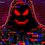# 'Special' Numbers

• $$0$$
• $1$

• $\sqrt2$
• $\phi \ or\ (\frac{\sqrt5+1}{2})$
• $i\ or\ \sqrt{-1}$

• $\tau\ or\ 2\pi$

• $\infty$ (Thanks to Jeff Giff)
• $W(1)$ (the solution to $xe^x=1$ (Thanks to James Watson)

• $299792458$ (the speed of light in a vacuum in $\frac{m}{s}$)
• $\approx 6.67408 \times 10^{-11}$ (the universal gravitational constant in $\frac{m^3}{kg \cdot s^2}$)
• $\approx 1.616255 \times 10^{-35}$ (the Planck length in $m$) (Thanks to James Watson)
• $\approx 5.39121\times 10^{-44}$ (the Planck time in $s$) (Thanks to James Watson)

If there are other numbers that you think should be added to this list, please comment.Note by Lâm Lê
10 months ago

This discussion board is a place to discuss our Daily Challenges and the math and science related to those challenges. Explanations are more than just a solution — they should explain the steps and thinking strategies that you used to obtain the solution. Comments should further the discussion of math and science.

When posting on Brilliant:

• Use the emojis to react to an explanation, whether you're congratulating a job well done , or just really confused .
• Ask specific questions about the challenge or the steps in somebody's explanation. Well-posed questions can add a lot to the discussion, but posting "I don't understand!" doesn't help anyone.
• Try to contribute something new to the discussion, whether it is an extension, generalization or other idea related to the challenge.

MarkdownAppears as
*italics* or _italics_ italics
**bold** or __bold__ bold
- bulleted- list
• bulleted
• list
1. numbered2. list
1. numbered
2. list
Note: you must add a full line of space before and after lists for them to show up correctly
paragraph 1paragraph 2

paragraph 1

paragraph 2

[example link](https://brilliant.org)example link
> This is a quote
This is a quote
    # I indented these lines
# 4 spaces, and now they show
# up as a code block.

print "hello world"
# I indented these lines
# 4 spaces, and now they show
# up as a code block.

print "hello world"
MathAppears as
Remember to wrap math in $$ ... $$ or $ ... $ to ensure proper formatting.
2 \times 3 $2 \times 3$
2^{34} $2^{34}$
a_{i-1} $a_{i-1}$
\frac{2}{3} $\frac{2}{3}$
\sqrt{2} $\sqrt{2}$
\sum_{i=1}^3 $\sum_{i=1}^3$
\sin \theta $\sin \theta$
\boxed{123} $\boxed{123}$

Sort by:

There are many numbers like c, g and more that you can add @Lin Le. C is the speed of light, and g is the universal gravitational constant.

$\infty$

- 10 months ago

$\Omega$ which is the solution to $xe^x=1$ (can also be expressed as $W(1)$ where $W(\cdot)$ is the Lambert's W Function/product log)

- 10 months ago

also the Planck length/time

- 10 months ago

How would you put Planck units as numbers?

- 10 months ago

normal units of measurement. for example: $1 \text{ Planck Length } \approx 1.616255×10^{-35} \text{ meters, }1 \text{ Planck Time } \approx 5.39 \times 10^{-44}\text{ seconds}$

- 10 months ago